Courses

# RD Sharma Solutions (Part - 1) - Ex-20.3, Mensuration - I, Class 7, Math Class 7 Notes | EduRev

## Class 7 : RD Sharma Solutions (Part - 1) - Ex-20.3, Mensuration - I, Class 7, Math Class 7 Notes | EduRev

The document RD Sharma Solutions (Part - 1) - Ex-20.3, Mensuration - I, Class 7, Math Class 7 Notes | EduRev is a part of the Class 7 Course RD Sharma Solutions for Class 7 Mathematics.
All you need of Class 7 at this link: Class 7

#### Question 1:

Find the area of a parallogram with base 8 cm and altitude 4.5 cm.

We have,
Base = 8 cm and altitude = 4.5 cm
Thus,
Area of the parallelogram = Base x Altitude
=  8 cm x 4.5 cm
= 36 cm

#### Question 2:

Find the area in square metres of the parallelogram whose base and altitudes are as under:
(i) Base = 15 dm, altitude = 6.4 dm
(ii) Base = 1 m 40 cm, altitude = 60 cm

We have,
(i) Base = 15 dm = (15 x 10) cm = 150 cm = 1.5 m                  [Since 100 cm  = 1 m]
Altitude = 6.4 dm = (6.4 x 10) cm = 64 cm = 0.64 m
Thus,
Area of the parallelogram = Base x Altitude
=  1.5 m x 0.64 m
= 0.96 m2

(ii) Base = 1 m 40 cm = 1.4 m             [Since 100 cm  = 1 m]
Altitude = 60 cm = 0.6 m
Thus,
Area of the parallelogram = Base x Altitude
=  1.4 m x 0.6 m
= 0.84 m2

#### Question 3:

Find the altitude of a parallelogram whose area is 54 dm2 and base is 12 dm.

We have,
Area of the given parallelogram = 54 dm2
Base of the given parallelogram = 12 dm
∴ Altitude of the given parallelogram  = Area/Base=54/12dm  = 4.5 dm

#### Question 4:

The area of a rhombus is 28 m2. If its perimeter be 28 m, find its altitude.

We have,
Perimeter of a rhombus = 28 m
∴ 4(Side) = 28 m                          [Since perimeter = 4(Side)]

⇒ Side = 28 m/4 =7 m
Now,
Area of the rhombus = 28 m2
⇒ (Side x Altitude) = 28 m2
⇒ (7 m x Altitude) = 28 m2

⇒ Altitude = 28 m2/ 7m =4 m

#### Question 5:

In Fig. 20, ABCD is a parallelogram, DL ⊥ AB and DM ⊥ BC. If AB = 18 cm, BC = 12 cm and DM = 9.3 cm, find DL.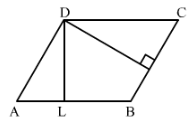We have,
Taking BC as the base,
BC = 12 cm and altitude DM = 9.3 cm
∴ Area of parallelogram ABCD = Base x Altitude
= (12 cm x 9.3 cm) = 111.6 cm2 ......... (i)
Now,
Taking AB as the base, we have,
Area of the parallelogram ABCD = Base x Altitude = (18 cm x DL).................(ii)
From (i) and (ii), we have

18 cm x DL = 111.6 cm2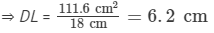#### Question 6:

The longer side of a parallelogram is 54 cm and the corresponding altitude is 16 cm. If the altitude corresponding to the shorter side is 24 cm, find the length of the shorter side.

We have,
ABCD is a parallelogram with the longer side AB = 54 cm and corresponding altitude AE = 16 cm.
The shorter side is BC and the corresponding altitude is CF = 24 cm.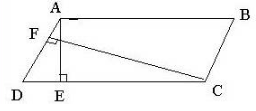Area of a parallelogram = base × height.We have two altitudes and two corresponding bases. So,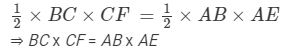Hence, the length of the shorter side BC AD = 36 cm.

#### Question 7:

In Fig. 21, ABCD is a parallelogram, DL ⊥ AB. If AB = 20 cm, AD = 13 cm and area of the parallelogram is 100 cm2, find AL.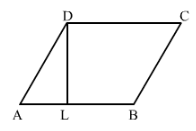We have,
ABCD is a parallelogram with base AB = 20 cm and corresponding altitude DL.
It is given that the area of the parallelogram ABCD = 100 cm2
Now,
Area of a parallelogram = Base x Height
100 cm2  = ABDL
100 cm2  = 20 cm x DL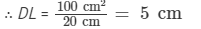Again by Pythagoras theorem, we have,
⇒ (13)2  = (AL)2 + (5)2
⇒ (AL)= (13)- (5)2

= 169 − 25 = 144
⇒ (AL)2 = (12)2
⇒ AL = 12 cm
Hence. length of AL is 12 cm.

#### Question 8:

In Fig. 21, if AB = 35 cm, AD = 20 cm and area of the parallelogram is 560 cm2, find LB.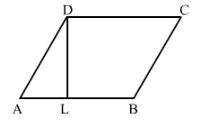We have,
ABCD is a parallelogram with base AB = 35 cm and corresponding altitude DL. The adjacent side of the parallelogram AD = 20 cm.
It is given that the area of the parallelogram ABCD = 560 cm2
Now,
Area of the parallelogram = Base x Height
560 cm2  = ABDL
560 cm2  = 35 cm x DL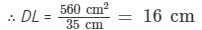Again by Pythagoras theorem, we have,
⇒ (20)2  = (AL)2 + (16)2
⇒ (AL)= (20)− (16)2

= 400 − 256 = 144
⇒ (AL)2 = (12)2
⇒ AL = 12 cm
From the figure,
AB = ALLB
35 cm = 12 cm + LB
LB = 35 cm − 12 cm
= 23 cm
Hence, length of LB is 23 cm.

#### Question 9:

The adjacent sides of a parallelogram are 10 m and 8 m. If the distance between the longer sides is 4 m, find the distance between the shorter sides.

We have,
ABCD is a parallelogram with side AB = 10 m and corresponding altitude AE = 4 m.
The adjacent side AD  = 8 m and the corresponding altitude is CF.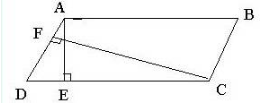Area of a parallelogram = Base × Height
We have two altitudes and two corresponding bases. So,
AD x CF = AB x AE
⇒ 8 m x CF = 10 m x 4 m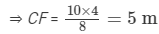Hence, the distance between the shorter sides is 5 m.

#### Question 10:

The base of a parallelogram is twice its height. If the area of the parallelogram is 512 cm2, find the base and height.

Let the height of the parallelogram be x cm.
Then the base of the parallelogram is 2x cm.
It is given that the area of the parallelogram = 512 cm2
So,
Area of a parallelogram =  Base x Height
512 cm2 = 2xx
512 cm22x2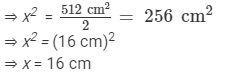Hence, base = 2x 2 x 16 = 32 cm and height = = 16 cm.

Offer running on EduRev: Apply code STAYHOME200 to get INR 200 off on our premium plan EduRev Infinity!

97 docs

,

,

,

,

,

,

,

,

,

,

,

,

,

,

,

,

,

,

,

,

,

,

,

,

,

,

,

,

,

,

;